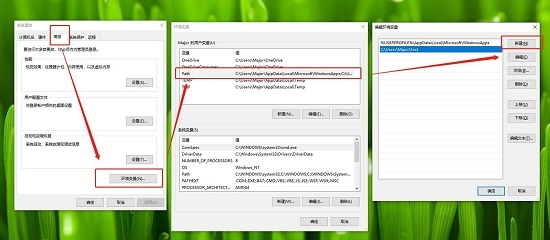# Pet绿色免费版 V1.1.32.0

50%

50%

• 软件介绍
• 软件截图

Pet绿色免费版是一款非常实用的办公工具。Pet绿色免费版它的功能十分简单，可以将键盘代替鼠标做一些常规操作，将工作快捷键在软件中进行设置，就可以使用键盘就能替代鼠标的操作，可以大大提高效率，感兴趣的朋友快来下载吧!

### 软件说明

键盘可以代替鼠标操作，打字的时候就可以更加专注。

快速命令模式，设置好后可以按【’+C】打开计算器。

提供了【Space+B】取色器功能。

帮助文件按【Space+Tab】查看

### 操作说明

操作都是基于长按空格后的操作

基础

Esc=退出

=重启 Shift+管理员身份重启

Tab=帮助

[=显示或隐藏托盘图标

]=设置或取消开机自启

光标移动

E=向上 Shift+E=向上多选 Ctrl+E=上一段

D=向下 Shift+D=向下多选 Ctrl+D=下一段

S=向左 Shift+S=向左多选 Ctrl+S=上一词

F=向右 Shift+F=向右多选 Ctrl+F=下一词

W=行首 Shift+W=选到行首 Ctrl+W=页首

R=行尾 Shift+R=选到行尾 Ctrl+R=页尾

页面操作

A=回车

G=菜单

CapsLock=取消

Q=退格

Z=撤销

X=剪切

C=复制

V=粘贴

Y=重做

多媒体

Up=调高音量

Down=调低音量

Left=播放上一首

Right=播放下一首

Home=暂停或继续播放

End=静音

取色器

B=显示鼠标区域色值 快速双击B=复制鼠标区域色值

笔记

P=快速打开本地笔记

鼠标操作

I=向上 Ctrl+I=高速 Shift+I=低速

K=向下 Ctrl+K=高速 Shift+K=低速

J=向左 Ctrl+J=高速 Shift+J=低速

L=向右 Ctrl+L=高速 Shift+L=低速

U=滚轮向上 Ctrl+U=向上翻页

O=滚轮向上 Ctrl+O=向下翻页

;=鼠标左键

H=鼠标右键

窗口

N=上个窗口

M=下个窗口

, =上个桌面(需要系统支持并且新建了桌面)

.=下个桌面(需要系统支持并且新建了桌面)

/=任务视图 Shfit+/=逆向切换(需要系统支持)

T=置顶或取消置顶当前活动窗口

特殊(不需要长按空格触发)

Win+CapsLock=启用或禁用增强模式(该模式不长按空格直接触发)

Win+Esc=禁用或启用所有功能

数字模式

按住Tab键右手就可以按数字键盘的布局输入数字

M=1, =2 .=3

J=4 K=5 L=6

U=7 I=8 O=9

N=0 H=+ Y=-

P== ;=* /=/

命令模式

按住'键后输入命令松开'键便可以运行命令

'键一般位于回车键左边一位

运行失败后会显示输入内容

例如按住'键后输入cmd打开命令提示符

### 注意事项

活动窗体属于管理员身份运行时热键暂时失效

比如活动窗体是任务管理器时就无法响应热键

办法是将本工具以管理员身份运行提升至相同的权限

设置开机自启目前都是非管理员身份运行

Pet绿色免费版 V1.1.32.0 软件截图
•• 本类周排行
• 本类总排行
• 本类推荐
• 热门标签